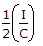# Electronics and Communication Engineering - Analog Electronics

46.

In a full wave diode rectifier circuit each diode carries current for

 A. 90° in each cycle B. 180° in each cycle C. 270° in each cycle D. 360° in each cycle

Explanation:

No answer description available for this question. Let us discuss.

47.

When a JFET is cut off, it's like an __________ switch and when it is saturated, it's like a __________ switch.

 A. closed, open B. open, closed C. open, open D. closed, closed

Explanation:

No answer description available for this question. Let us discuss.

48.

The mobility of e- for Ge and Si respectively is

 A. 1.66, 2.5 B. 2.5, 1.66 C. 2.7, 2.33 D. 2.33, 2.7

Explanation:

No answer description available for this question. Let us discuss.

49.

If a capacitor C is charged by a constant current I, then the rate of change of voltage with time given by sweep speed equal to

 A. C/I B. IC C. I/C D.Explanation:

No answer description available for this question. Let us discuss.

50.

In operational Amplifier, Common mode voltage gain should be

 A. 0 B. ∞ C. 1 D. 0.5